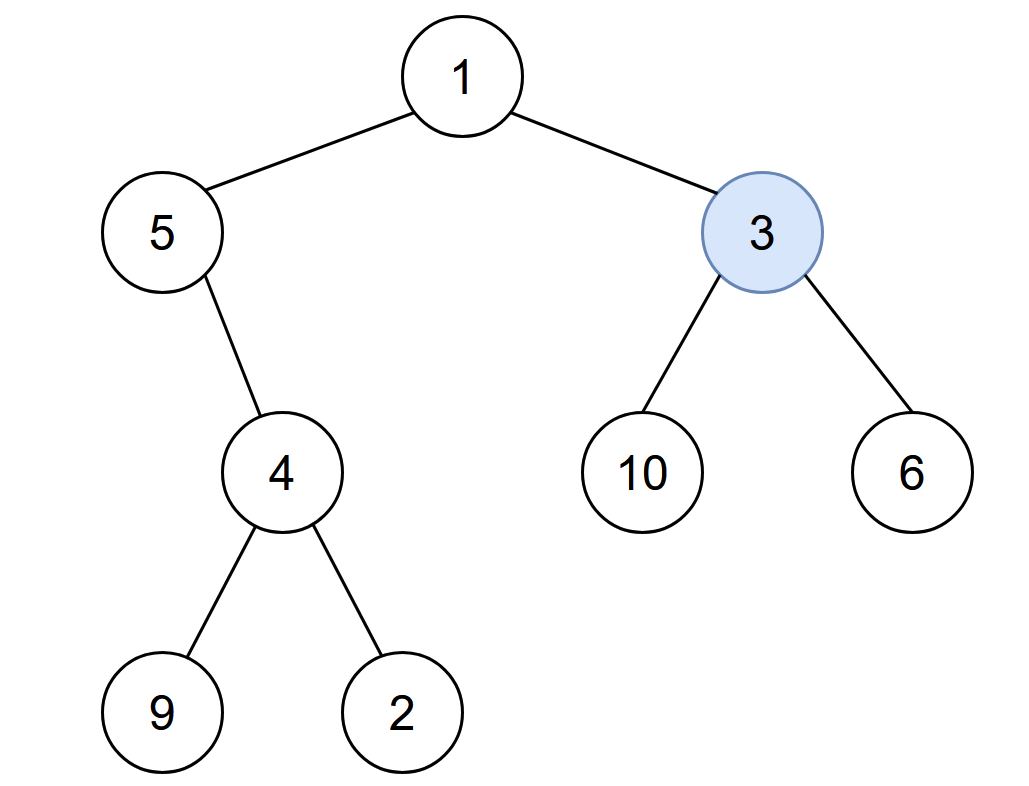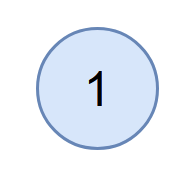# 2385. Amount of Time for Binary Tree to Be Infected | Leetcode Solution

You are given the `root` of a binary tree with unique values, and an integer `start`. At minute `0`, an infection starts from the node with value `start`.

Each minute, a node becomes infected if:

• The node is currently uninfected.
• The node is adjacent to an infected node.

Return the number of minutes needed for the entire tree to be infected.

Example 1:```Input: root = [1,5,3,null,4,10,6,9,2], start = 3
Output: 4
Explanation: The following nodes are infected during:
- Minute 0: Node 3
- Minute 1: Nodes 1, 10 and 6
- Minute 2: Node 5
- Minute 3: Node 4
- Minute 4: Nodes 9 and 2
It takes 4 minutes for the whole tree to be infected so we return 4.
```

Example 2:```Input: root = , start = 1
Output: 0
Explanation: At minute 0, the only node in the tree is infected so we return 0.
```

Constraints:

• The number of nodes in the tree is in the range `[1, 105]`.
• `1 <= Node.val <= 105`
• Each node has a unique value.

### A node with a value of `start` exists in the tree.Solution:

The solution to this question is straightforward. If you know graphs, you can answer this question in less than a minute. We can app DFS and can get the answer, but how we can do that as this is a tree? Either we can make a new graph with this tree or we can traverse the tree once and then store the parent node of all the nodes. Then we can simply apply DFS on this.

1.#include
using namespace std;

int main(){

cout<<"Welcome to FCFS \n";
cout<<"Enter the number of Processes: ";
int k=1;
cin>>k;
int pid,arrivalTime,burstTime;
vector>process;
while(k--){
cout<<"Enter Process ID: ";
cin>>pid;
cout<<"Enter Arrival Time: ";
cin>>arrivalTime;
cout<<"Enter the Burst Time: ";
cin>>burstTime;
process.push_back({pid,arrivalTime,burstTime});
}
int st=0;
cout<<"Process No: Arrival Time: Burst Time: Completion Time: Turn Around Time: Waiting Time: Response Time:\n";
for (int i = 0; i < process.size(); i++)
{
vectorv=process[i];
int ct=max(v+v,st+v);
cout<<v<<" "<<v<<" "<<v<<" "<<ct<<" "<<ct-v<<" "<<abs(st-v)<<" "<<max(0,st-v)<<endl;
st=ct;
}

return 0;
}

If you have any doubts/suggestion/any query or want to improve this article, you can comment down below and let me know. Will reply to you soon.